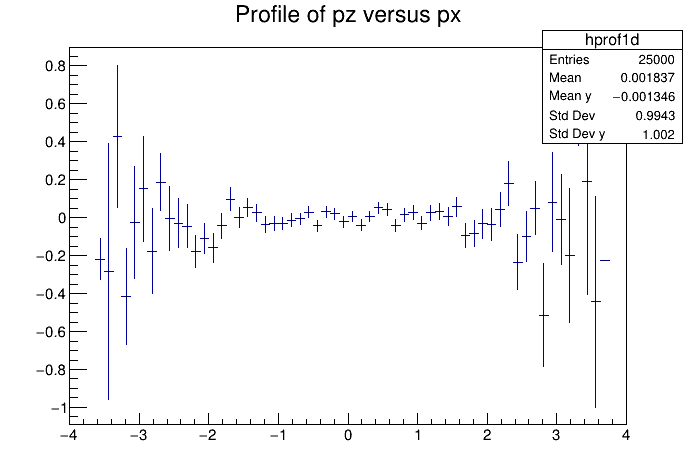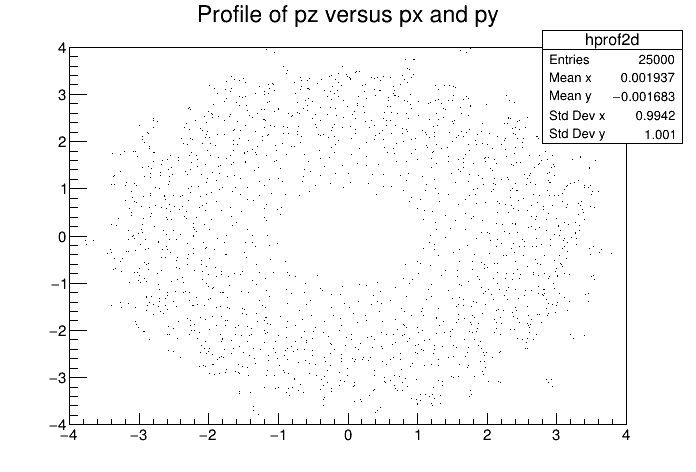# Df 0 0 3_Profiles¶

This tutorial illustrates how to use TProfiles in combination with the RDataFrame. See the documentation of TProfile and TProfile2D to better understand the analogy of this code with the example one.

Author: Danilo Piparo
This notebook tutorial was automatically generated with ROOTBOOK-izer from the macro found in the ROOT repository on Monday, July 06, 2020 at 11:29 AM.

In :
import ROOT

Welcome to JupyROOT 6.23/01


A simple helper function to fill a test tree: this makes the example stand-alone.

In :
def fill_tree(treeName, fileName):
d = ROOT.RDataFrame(25000)
d.Define("px", "gRandom->Gaus()")\
.Define("py", "gRandom->Gaus()")\
.Define("pz", "sqrt(px * px + py * py)")\
.Snapshot(treeName, fileName)


We prepare an input tree to run on

In :
fileName = "df003_profiles_py.root"
treeName = "myTree"
fill_tree(treeName, fileName)


We read the tree from the file and create a RDataFrame.

In :
columns = ROOT.vector('string')()
columns.push_back("px")
columns.push_back("py")
columns.push_back("pz")
d = ROOT.RDataFrame(treeName, fileName, columns)


Create the profiles

In :
hprof1d = d.Profile1D(("hprof1d", "Profile of pz versus px", 64, -4, 4))
hprof2d = d.Profile2D(("hprof2d", "Profile of pz versus px and py", 40, -4, 4, 40, -4, 4, 0, 20))


And Draw

In :
c1 = ROOT.TCanvas("c1", "Profile histogram example", 200, 10, 700, 500)
hprof1d.Draw()
c2 = ROOT.TCanvas("c2", "Profile2D histogram example", 200, 10, 700, 500)
hprof2d.Draw()


Draw all canvases

In :
from ROOT import gROOT
gROOT.GetListOfCanvases().Draw()